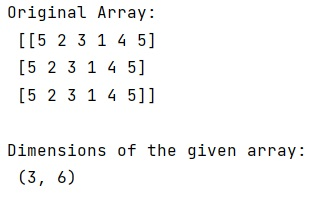# How to find the length (or dimensions, size) of a NumPy matrix?

Learn, how to find the length (or dimensions, size) of a NumPy matrix in Python?
Submitted by Pranit Sharma, on December 26, 2022

NumPy is an abbreviated form of Numerical Python. It is used for different types of scientific operations in python. Numpy is a vast library in python which is used for almost every kind of scientific or mathematical operation. It is itself an array which is a collection of various methods and functions for processing the arrays.

## Finding the length (or dimensions, size) of a NumPy matrix

Suppose we are given a NumPy matrix and we need to find the length of a row (or column) of this matrix.

Numpy provides us an efficient way called array.shape() which is a property of both NumPy array ndarray's and matrices.

This method will return a tuple that contains the number of words and number of columns and also, NumPy provides us a method called matrix.size() which returns the number of elements in the array.

Let us understand with the help of an example,

## Python code to find the length (or dimensions, size) of a NumPy matrix

```# Import numpy
import numpy as np

# Creating a numpy array
arr = np.array([
[5, 2, 3, 1, 4, 5],
[5, 2, 3, 1, 4, 5],
[5, 2, 3, 1, 4, 5]
])

# Display original arrays
print("Original Array:\n",arr,"\n")

# Using arr.shape method to find the size
res = arr.shape

# Display result
print("Dimensions of the given array:\n",res)
```

Output: